# Ncert solutions for Fractions exercise 7.1 class 6 Maths Ncert

Ncert Maths Class 6 Chapter 7 exercise 7.1 problems with solutions are given below.

Study the textbook lesson Fractions very well.

Observe the example problems and solutions given in the textbook. Try them.

Observe the given below solutions and try them in your own method

You can see

Ncert solutions for class 6 maths

Fractions

Exercise 7.1

Exercise 7.2

Exercise 7.3

M

# 6th class chapter 7 exercise 7.1 Fractions solutions Ncert Maths

Chapter 7 – Fractions

Exercise 7.1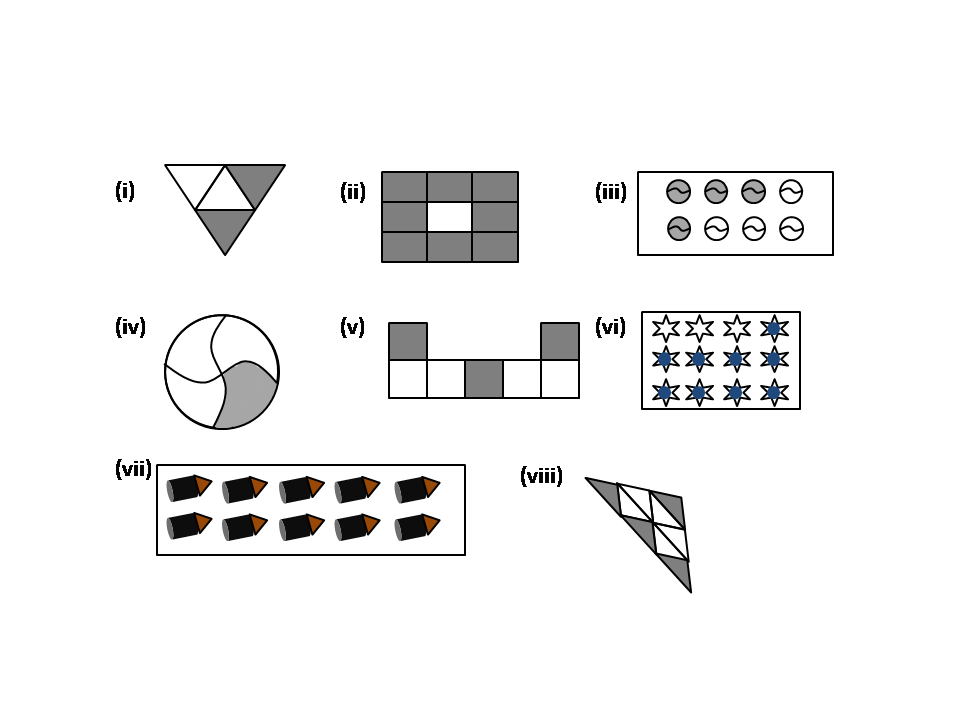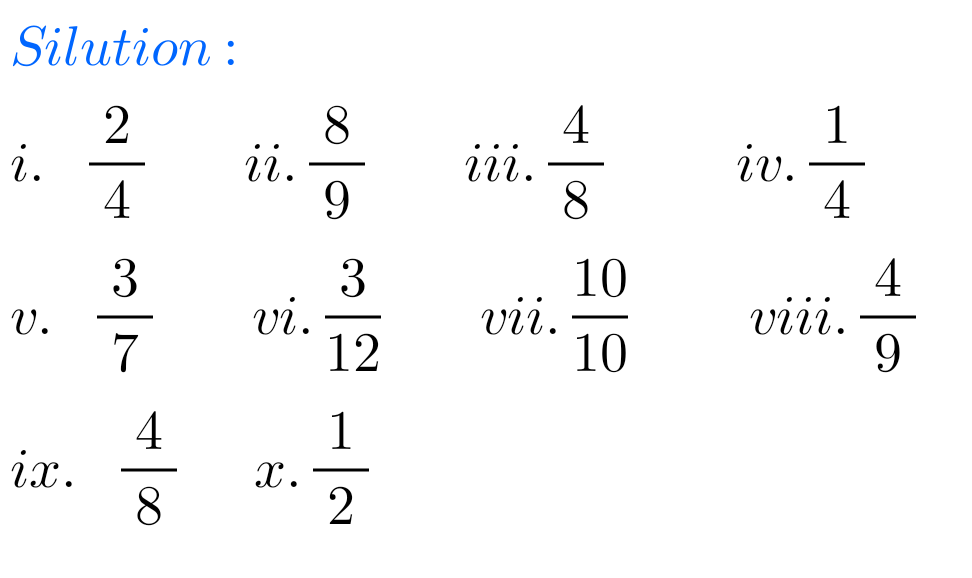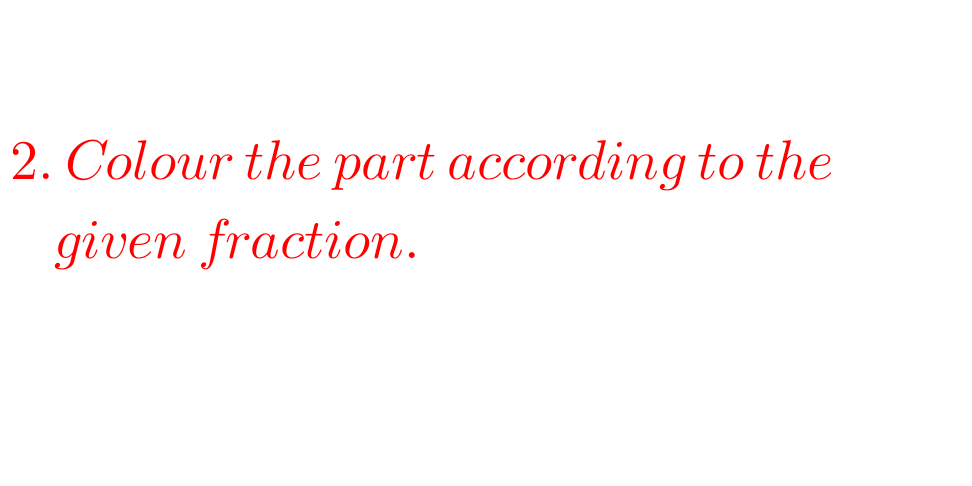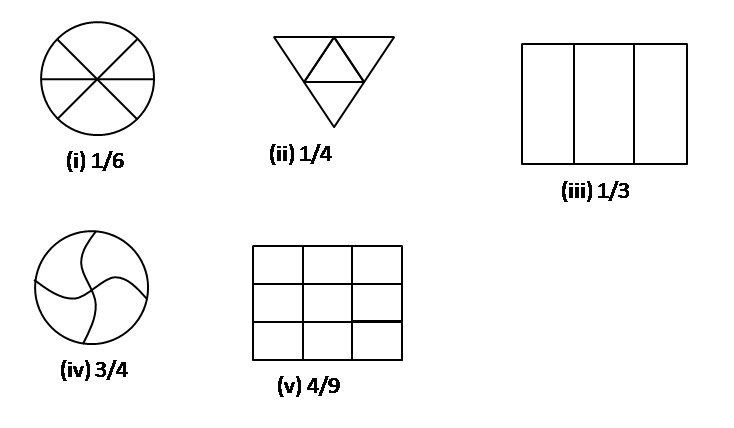Solution :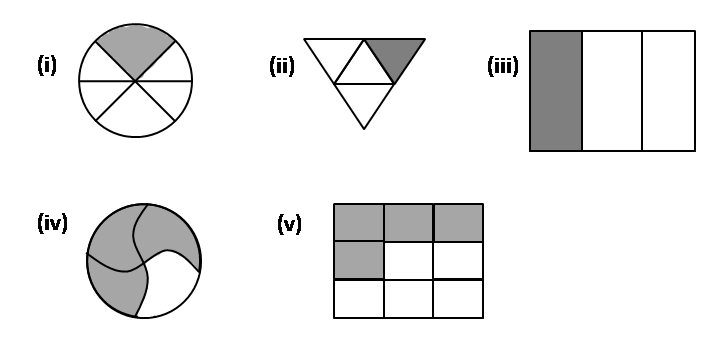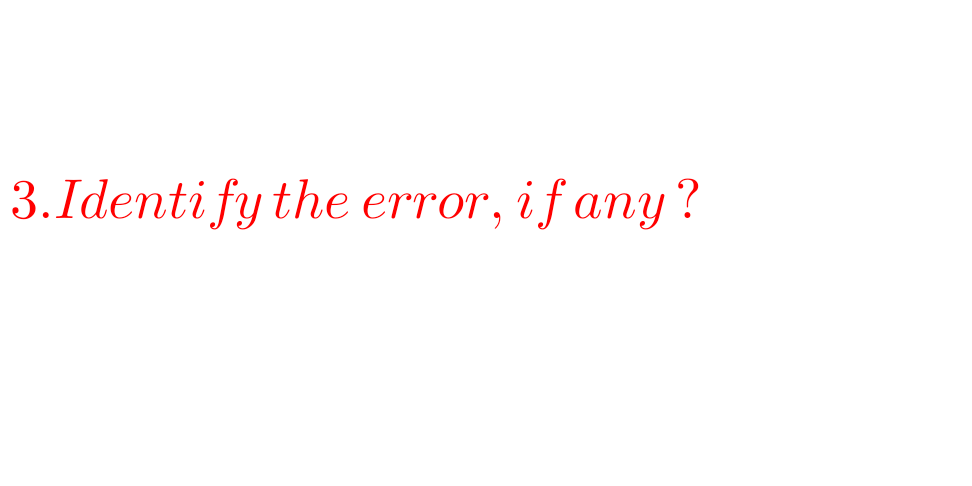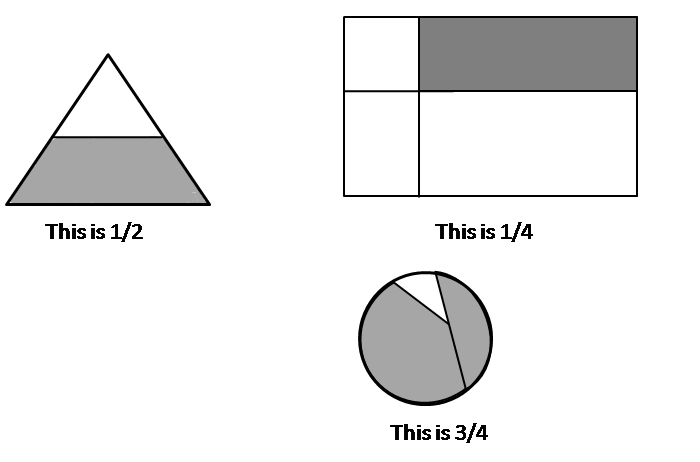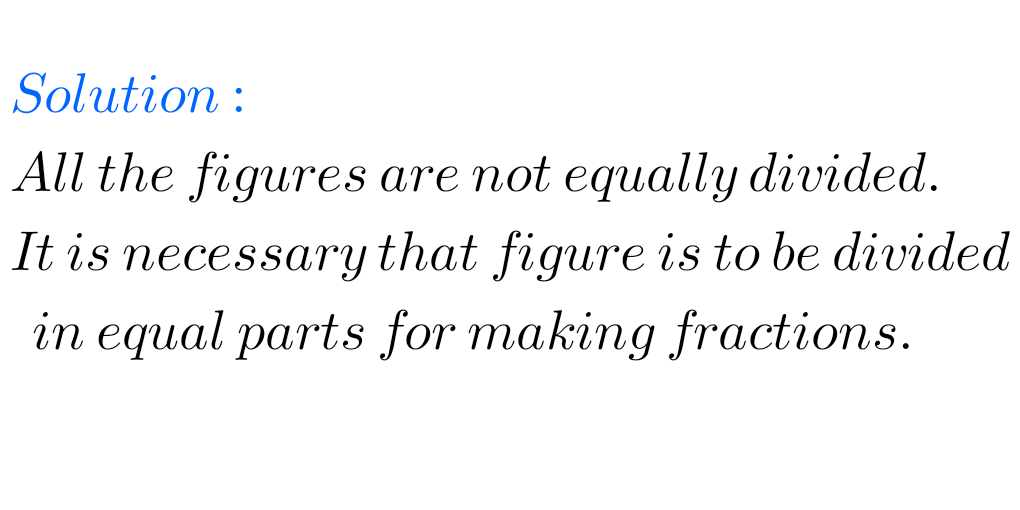## Exercise 7.1 Ncert Maths class 6 solutions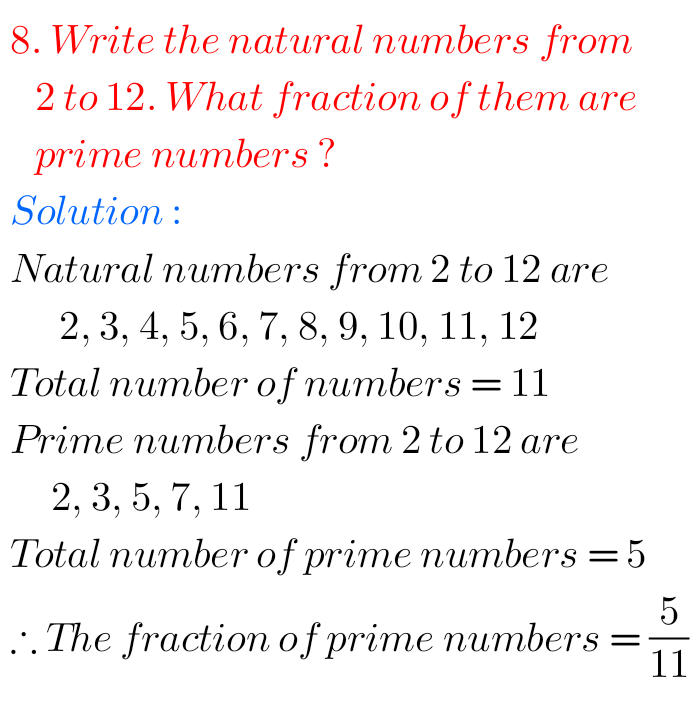## Solutions for Fractions Ncert Maths exercise 7.1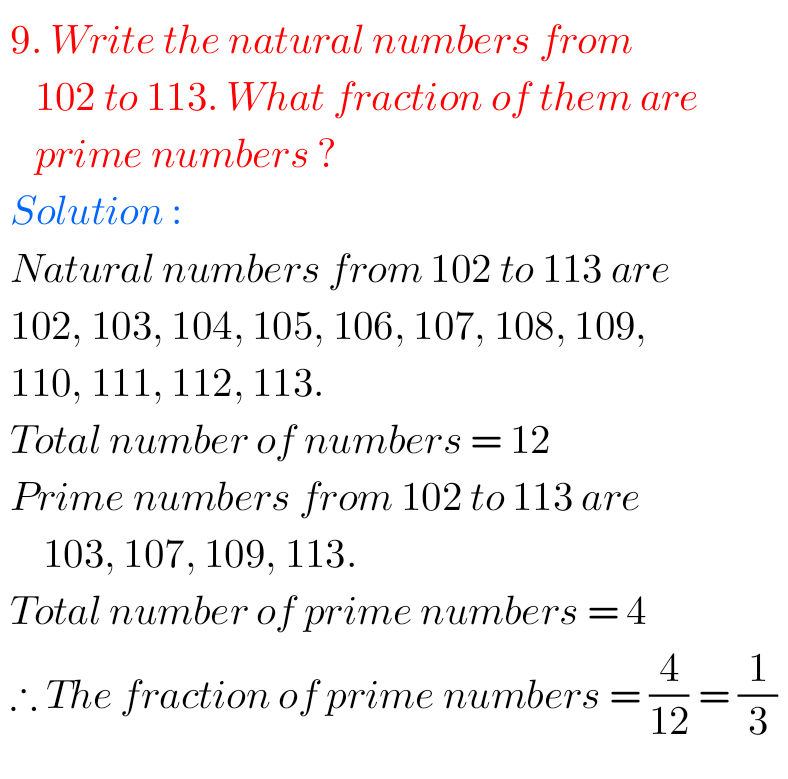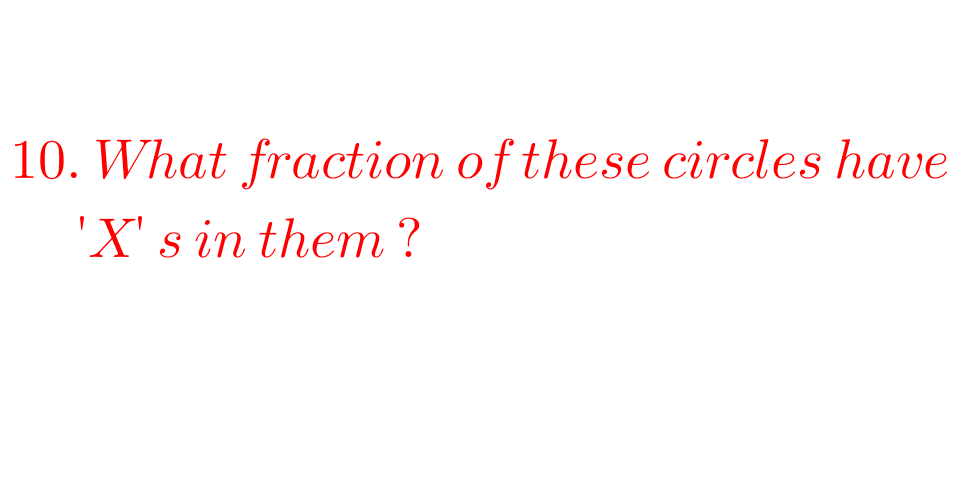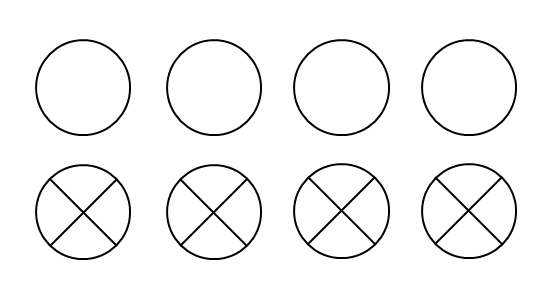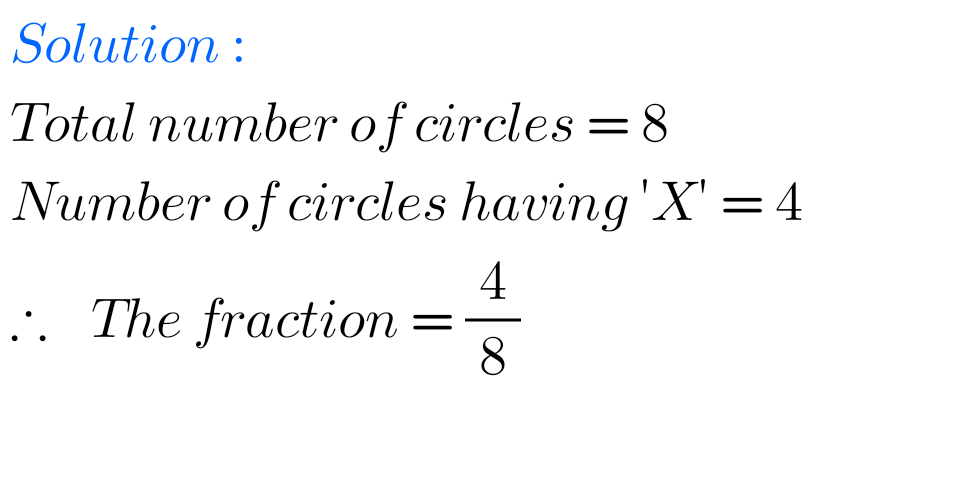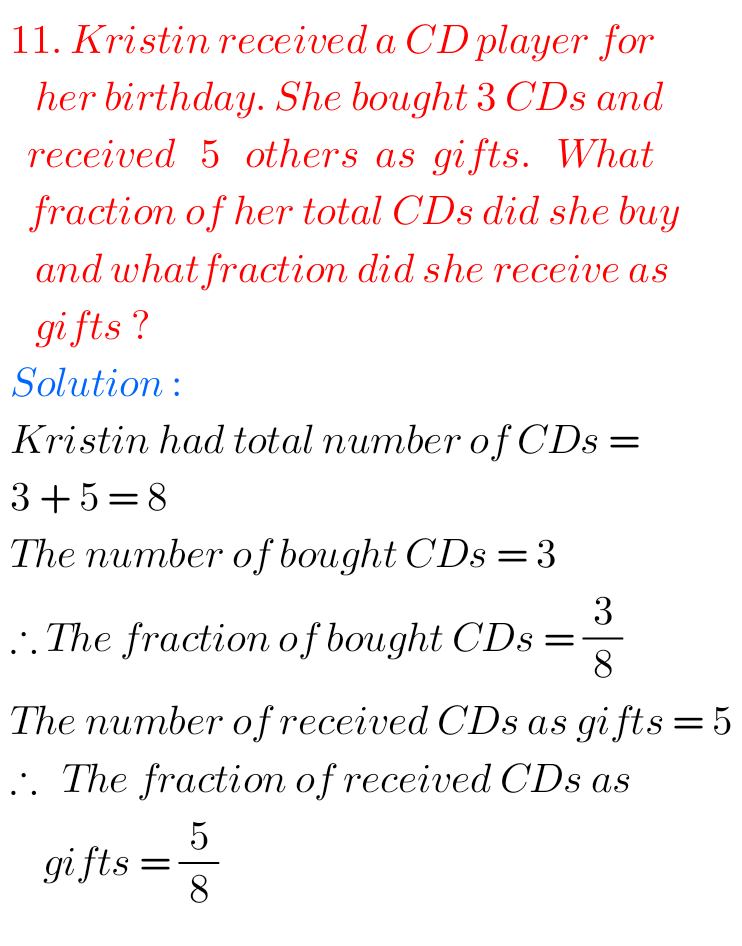Note : Observe the solutions and try them in your own method.